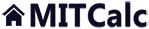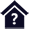#Vehicle aerodynamics

Clicking on an item from the content will take you to the relevant paragraph.

## Vehicle aerodynamics

The program is intended for simple simulation of vehicle motion. The program solves the following tasks:

1. Simple definition of vehicle parameters.
2. Selection from a list of typical vehicles.
3. Calculation of forces.
4. Calculation of the load, energy, consumption and travel distance.
5. Graphic representation of the results.

Data, procedures, algorithms and information from professional literature and the catalogues of the manufacturers are used in the calculation.

User interface.

Purchase, Price list.

## Control, structure and syntax of calculations.

Information on the syntax and control of the calculation can be found in the document "Control, structure and syntax of calculations".

## Information on the project.

Information on the purpose, use and control of the paragraph "Information on the project" can be found in the document  "Information on the project".

## Theory.

The following formulas are used in the calculation:

#### Air pressure related to the elevation:

p [kPa] = p0 / (1 - (0.0065 * h) / (t+0.0065 * h + 273.15)) (-5.257)

p0 ... Air pressure at sea level (101.32) [kPa]
h ..... Altitude [m]
t ...... Temperature [°C]

#### Air density:

Q [kg/m^3] = (p * 1000 * 1 * 0.02895) / (8.314 * (273 + t))

#### Aerodynamic resistance:

Fa [N] = 0.5 * Q * cx * S * v^2

cx ... Coefficient of resistance [~]
S ... Facing [m^2]
v ... Vehicle speed [m/s]

#### Tyre rolling resistance (kola):

Fr [N] = m * 9.81 * fr / (dw / 2)

fr ..... Traction coefficient [~]
m ..... Vehicle weight [kg]
dw ... Tyre (wheel) diameter [m]

#### Climbing / descending force (motion on an inclined plane):

Fu [N] = sin(alfa) * m * 9.81

alfa ... Climbing / descent angle [rad]
m ..... Vehicle weight [kg]

#### Wheel power:

Pw [kW] = (Fa + Fr + Fu) * v / 1000

v ... Speed [m/s]

## Process of calculation.

1. Select calculation units and environmental parameters [1.0].
2. Select any of the sample examples [2.1], or define own vehicle parameters [2.2-2.20].
3. Enter the speed [2.22].

## Setting of environment units and parameters. 

In this paragraph, select the units, temperature and altitude.

### 1.1 Calculation units.

Select the desired calculation unit system from the selection list. Upon switching units, all the values shall change immediately.

### 1.5 Air density.

Upon picking the button, you can enter any value independent of [1.2-1.3].

## Calculation of the vehicle motion and parameters. 

In this paragraph, select the vehicle parameters, speed and ascertain the drive performance parameters.

### 2.1 Vehicle parameters.

From the selection list, pick the sample vehicle. If you will modify the vehicle parameters, select the corresponding drive type (combustion engine / electric motor). The parameters of further calculation and results are set on the basis of this choice. To define your own values, select the button on the line [2.2].

### 2.5 Coefficient of reduction of the facing.

The real facing differs from the rough facing s=w*h. For simpler calculation, this coefficient has been introduced, which allows quick estimate of the real facing for various types of vehicles from their tabular width and height.

### 2.14 Energy in 1kg of fuel / Energy in 1kg of the batteries.

Depending on the processing methods and manufacturers, it is in the range:

Petrol: 12.1-12.9 kWh/kg
Diesel: 11.6-11.9 kWh/kg

Battery:
LiIon: 0.14 - 0.2 kWh/kg
LiFePO4: 0.12 - 0.16 kWh/kg

### 2.16 Maximum engine power.

For the sample vehicle from the table, the values are obtained from the manufacturers’ data. The entered value has an effect on the calculation of the top speed for [2.23].

### 2.17 Engine efficiency.

The values may differ substantially depending on the type of engine (petrol, diesel, electric motor), environment and loading mode. For the sample vehicles from the table, these values are estimated on the basis of the manufacturer’s declared fuel consumption, performance diagrams, travel distances, etc.

### 2.18 Efficiency of the gearing, bearings…

Depends on the design of the drive. For the vehicles from the table, the values are estimated from available literature and the manufacturers’ catalogues.

### 2.23 Vehicle speed.

Enter the vehicle speed. The vehicle top speed is calculated by pressing the button [v: v = v max].

### 2.25 Traction coefficient.

Determines the rolling resistance of the wheels. Typical examples are given in the selection list. Enter an own value after checking the button at [2.26].

### 2.28 Climbing / descent.

Enter the climbing angle (descent...negative value) of the path along which the vehicle is moving. The button on the right is used to:

[a: a = 0] ... Set the angle at 0

[a: Fu = -Fr] ... Set such an angle for the descent to be equal to the rolling resistance force

[a: Fu = -Fr-Fa] ... Set such an angle achieve the entered speed [2.23] without using the engine (Pwe = 0)

### 2.33 Maximum acceleration.

The maximum acceleration available for the specific vehicle. It depends mainly on the transmission of the driving force to the road, the drive type, the tires, the structure, etc.

Dragsters ... up to 5 g
Supersports ... up to 1.5 g
Regular vehicles ... 0.2 - 0.8 g

### 2.34 Theoretical time to achieve the specified speed

The theoretical time achievable provided an ideal constant power is delivered for the period of acceleration under ideal conditions. In the calculation, the only limitation considered is the maximum acceleration [2.33]. (Limited transfer of the power from the tire to the road surface.)

### 2.46 Graph I.

Allows display of the parameters in relation to the speed. Select the scope of the graph from the selection list above the graph, and then select the desired parameters to be displayed from the list on the left and right. The speed from the calculation is indicated by a red line.

### 2.47 Graph II.

It shows the acceleration and the speed as a function of time.
The graph range can be set by selecting the range above the graph.

## Setting calculations, change the language.

Information on setting of calculation parameters and setting of the language can be found in the document "Setting calculations, change the language".

## Workbook (calculation) modifications.

General information on how to modify and extend calculation workbooks is mentioned in the document "Workbook (calculation) modifications".